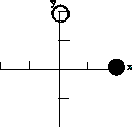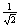Teaching Physics with the Physics Suite Edward F. Redish

Finding the E field

 The picture on the right displays two charges placed on a coordinate grid. Each of the tic marks on the axes represents 1 m. The magnitude of the charge represented by the solid circle is q1 and is at the position (2,0), while the charge represented by the open circle is q2 and is at the position (0,2). On the left below is a list of 5 sets of configurations [labeled i)-v)] specifying the value of the charges and a positions at which the E field is to be measured. For ease of calculation, these are represented in terms of the Coulomb constant K = 1/4pe0. On the right is a list of twelve possible electric fields represented as x-y components. For each of the four configurations select the E-field components which represent the field that would be found at that position.Configuration

Possible E-fields

 q1 q2 Position to test the E field i) 4/K -4/K (0,0) ii) 4/K -4/K (2,2) iii) 4/K 0 (0,0) iv) 0 -8/K (-2,2) v) 0 -8/K (2,0)
 (a) (1, 0) (g)(1,-1) (b) (0, -1) (h)(-1,1) (c) (1, 1) (i) 2 (1, 0) (d) (-1, 0) (j) 2 (0, 1) (e) (-1, 1) (k) 2 (-1, 0) (f) (0, 1) (l) none of the above

Not finding what you wanted? Check the Site Map for more information.